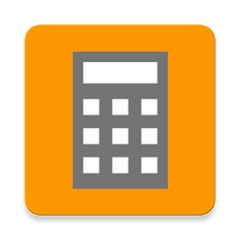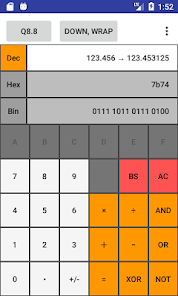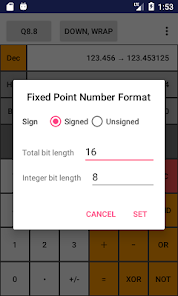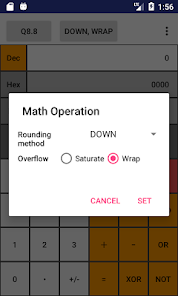# FixedPointCalc

500+EveryoneThe calculator for hardware engineers who uses a fixed point number in FPGA and so on.

* When you input a real number, it's converted to a fixed point number(hexadecimal, binary number).
* When you input a fixed point number, it's converted to a real number.
* Any fixed point number formats are available.
* You can set the rounding mode and overflow mode(Wrap around or Saturation).
* The four basic arithmetic operations and logical operations are available.

How to use:
* Set the fixed point number format. Tap the button on the upper left to set the signed or unsigned, the total bit length and the integer bit length.
* The format represents as Q format. Qm.f means m integer bits and f fractional bits.
* UQm.f means unsigned value.

* Tap the button on the upper right to set the rounding mode and overflow mode.
* Rounding modes are:
* UP: Rounding mode where positive values are rounded towards positive infinity and negative values towards negative infinity.
* DOWN: Rounding mode where the values are rounded towards zero.
* CEILING: Rounding mode to round towards positive infinity.
* FLOOR: Rounding mode to round towards negative infinity.
* HALF_UP: Rounding mode where values are rounded towards the nearest neighbor. Ties are broken by rounding up.
* HALF_DOWN: Rounding mode where values are rounded towards the nearest neighbor. Ties are broken by rounding down.
* HALF_EVEN: Rounding mode where values are rounded towards the nearest neighbor. Ties are broken by rounding to the even neighbor.

* Over flow modes are:
* Saturate: Do the saturate calculation.
* Wrap around: The overflowed bits are discarded.

* Select the radix by tapping Dec, Hex and Bin.
* Dec: You can input a real number. During input, the input value is displayed on the left side of the arrow and the value rounded to the fixed decimal point is displayed on the right side.
* Hex: You can input a hexadecimal number in fixed point number format.
* Bin: You can input a binary number in fixed point number format.

* AC key clears calculation.
* BS key means 'back space'.
* Multiplication and division takes precedence over addition and subtraction. So 1 + 2 x 3 = 7.
Updated on
Aug 22, 2022

## Data safety

Safety starts with understanding how developers collect and share your data. Data privacy and security practices may vary based on your use, region, and age. The developer provided this information and may update it over time.No data shared with third partiesNo data collected